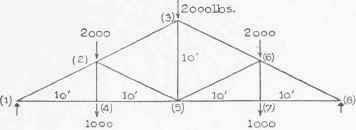Examples. 1. It is required to construct a stress diagram for the truss represented in Fig. 24 supported at its ends and sustaining three loads of 2,000 pounds as shown. Evidently the reactions equal 3,000 pounds.

Following the directions of Art. 2G, we letter the truss diagram, then draw the polygon for the loads and reactions. Thus, to the scale indicated in Fig. 24 (5), AB, BC, and CD represent the loads at joints (2), (3) and (5) respectively and DE and EA represent the right and the left reactions respectively. Notice that the polygon (ABCDEA) is a clockwise one.

At joint (l)there are three forces, the left reaction and the forces exerted by the members af and fe. Since the forces exerted by these two members must be marked AF and EF we draw from A a line parallel to af and from E one parallel to ef and mark their intersection F. Then EAFE is the polygon for joint (1), and since EA acts up (see the polygon), AF acts down and FE to the right. We, therefore, place the proper arrowheads on of and fe near (1). and record (see adjoining table) that the stresses in those members are compressive and tensile respectively. Measuring, we find that AF and FE equal 6,150 and 5,100 pounds respectively.

 Member. af fe bg fg gh Stress.. - 6,150 + 5,100 - 4,100 - 1,875 + 2,720

We may next draw the polygon for joint (6) or (2) since there are but two unknown forces at each. At joint (2) for instance, the unknown forces are those exerted by fg and bg, and the known are the load ah (2,000 pounds) and the force exerted by of. Since the unknown forces must be marked FG and GB, draw from F a line parallel to fg, from B a line parallel to bg, and mark their intersection G. Then the polygon for the joint is FABGF, and since AB acts down (see the polygon) BG and GF act down and up respectively. Therefore, place the proper arrowheads on bg and gf near (2), and record that the stresses in those members are both compressive. Measuring, we find that BG and GF scale 4,100 and 1,875 pounds respectively. Now draw a polygon for joint (3) or (6) since there are but two unknown forces at each joint. At (3) for instance, the unknown forces are those exerted by ch and gh, the known forces being the load (2,000 pounds) and the force 4,100 pounds, exerted by bg. Since the unknown forces must be marked CH and GH, draw from C a line parallel to ch, from G one parallel to gh, and mark their intersection H. Then GBCHG is the polygon for the joint, and since BC acts down (see the polygon) CH acts up and HG down. Therefore, place the proper arrowheads on ch and hg near (3), and record that the stresses in those members are compressive and tensile respectively. Measuring, we find that CH and HG scale 4,100 and 2,720 pounds respectively.Fig. 25.

It is plain that the stress in any member on the right-hand side is the same as that in the corresponding member on the left, hence it is not necessary to construct the complete stress diagram.

2. It is required to analyze the truss of Fig. 25 which rests on end supports and sustains three loads each of 2,000 pounds as shown. Each member is 1G feet long.Fig. 26.

Evidently, reactions are each 3,000 pounds. Following directions of Art. 26, first letter the truss diagram and then draw a polygon for the loads and reactions representing them in either order in which they occur about the truss. DCBAED is a counter-clockwise polygon, DC, CB, and BA representing the loads at joints (1), (2) and (3), AE the left reaction, ED the right reaction.Fig. 27.

The construction of the polygons is carried out as in the preceding illustration, and little explanation is necessary. The polygon for joint (4) is AEFA, EF (1,725 pounds tension) representing the stress in ef and FA (3,450 pounds compression) that in of. The polygon for joint (3) is BAFGB, FG (1,150 pounds tension) representing the stress in fg and GB (2,300 pounds compression) that in gb. The polygon for joint (5) is GFEIIG, EH (2,875 pounds tension) representing the stress in eh and HG (1,150 pounds compression) that in hg.

Evidently the stress in any member on the right side of the truss is like that in the corresponding member on the left, therefore it is not necessary to construct the remainder of the stress diagram.

## Examples For Practice

1. Analyze the truss represented in Fig. 26, it being supported at its ends and sustaining three loads of 2,000 and two of 1,000 pounds as represented.

 - - .-. - .- - - Member.. 12 23 14 45 24 25 35 Stress... -8,950 -5,600 +8,000 +8,000 +1,000 -3,350 +3,000

2. Analyze the truss represented in Fig. 27, it being supported at its end's and sustaining five 2,000-pound loads and two of 1,000 as shown.

 STRESS RECORD. __. __ __ __ __ .__ __ .__ Member. 12 23 34 51 52 53 54 56 Stress . -11.200 -8,900 -8,900 +10,000 -2,000 -2,000 +3,600 +6,000